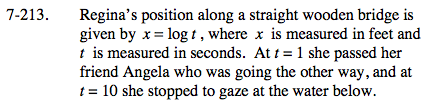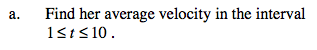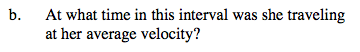### Home > CALC > Chapter Ch7 > Lesson 7.4.4 > Problem7-213

7-213.$\text{average velocity}=\frac{\text{displacement}}{\text{time}}$This is an application of the Mean Value Theorem, which guarantees that, on a closed interval, IROC = AROC somewhere if the function is continuous and differentable.